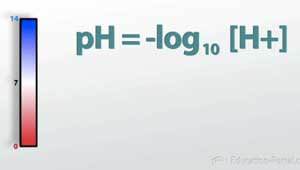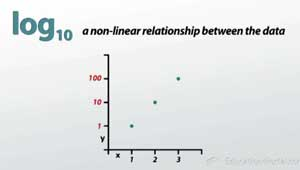CLEP Biology Test Prep / Course / Chapter

# Acids and Bases

Lesson Transcript
Instructor: Zach Pino
Acids are substances that contribute molecules, while bases are substances that can accept them. Learn how to define acids and bases, explore the pH scale, and discover how to find pH values. Updated: 10/24/2021

## The pH ScaleThis type of scale is called a pH Scale. This is basically just a very simplistic way that we could express measurements of the acidity of a solution. In science, we want to have more concrete measurements of things; we don't want to just put it arbitrarily in this continuum, we want to associate an actual number with this acidity.An error occurred trying to load this video.

Try refreshing the page, or contact customer support.

Coming up next: The Laws of Thermodynamics

### You're on a roll. Keep up the good work!

Replay
Your next lesson will play in 10 seconds
• 0:10 The pH Scale
• 0:38 Finding pH Values
• 1:15 Logarithmic Equations
• 3:32 Defining an Acid
• 5:30 Defining a Base
• 7:02 Lesson Summary
Save Timeline
Autoplay
Autoplay
Speed Speed

## Finding pH Values

So pH is defined as the negative log of the concentration of hydrogen ions. So what does that mean? It sounds really complicated, right?

Let's start with this right here first. So this part of the equation (H+) is just defining concentration of hydrogen ions. A hydrogen ion, if you recall, is basically just a hydrogen atom which has lost one of its electrons. We'll come back to this in a minute and talk a little bit more specifically about how this relates.

## Logarithmic Equations

This part of the equation then is going to tell us what kind of relationship the pieces of data have between each other. What we have when we talk about a log scale is we have a non-linear relationship between the data, and every time I move a unit on the x scale, I am moving ten times as much as the previous y unit.

The difference between y values increases exponentially - or logarithmically - every time I increase the x value by one.

What exactly is this part of the log part of the equation doing? What does that mean? To put this into terms of a scale that you might be familiar with that's a log scale, think about the Richter Scale. The Richter Scale measures the intensity of earthquakes. If I have an earthquake that is 4.0 on the Richter Scale, that has a certain power that's associated with it. If I talk about an earthquake that's 5.0, it's not that it was just one unit stronger than the 4.0 earthquake, it's actually 10 times more powerful than the 4.0 earthquake. And then if we talk about something that's 6.0, that's 10 times more than the 5.0 earthquake and 100 times more than the 4.0 earthquake. You can see then that by using a log scale, it allows us to measure a wider range of values.So for instance, water has a concentration of hydrogen ions of 10^-7.

So you can see here that we're going to be measuring very small values, and because we're usually dealing with concentration values that are less than one, we want to use this negative sign to make everything positive.

## Defining an Acid

Let's go back to our pH scale here, and by using this equation, what that means is that water is going to translate to a pH of 7. Anything that is more than 7, we're going to call a base; anything that is less than 7, we're going to call an acid. We define these numbers because of the way these types of molecules behave in terms of the hydrogen ion concentration. So let's revisit this hydrogen ion concentration concept a little more closely.

Let's talk about acids first. An acid is defined as a molecule which donates hydrogen ions. Let's take an example of a strong acid known as hydrogen chloride. Hydrogen chloride, because it's a strong acid, will completely dissociate and it's going to form one molecule of hydrogen ion and also one chlorine ion. Because it donates into solution this hydrogen ion, this molecule, hydrogen chloride, is known as an acid.

To unlock this lesson you must be a Study.com Member.

### Register to view this lesson

Are you a student or a teacher?

Back

### Resources created by teachers for teachers

Over 30,000 video lessons & teaching resources‐all in one place.Video lessonsQuizzes & WorksheetsClassroom IntegrationLesson Plans

I would definitely recommend Study.com to my colleagues. It’s like a teacher waved a magic wand and did the work for me. I feel like it’s a lifeline.

Jennifer B.
TeacherCreate an account to start this course today
Used by over 30 million students worldwide